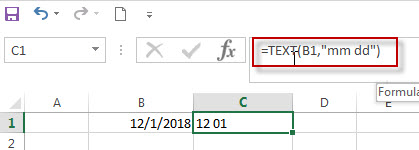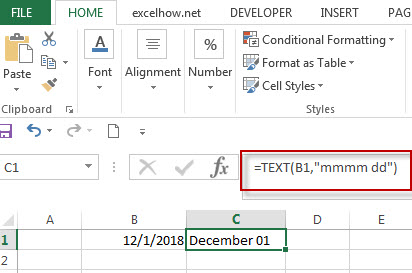# Convert date to month and day only in excel

In excel, how to get the month and day string from an excel date value. How to convert the dates to text string, just only display as month and day. This post will guide you how to convert date to month and day value only using Text Function in Microsoft Excel.

## Convert date to month and day with Text Function

If you want to convert the date (mm/dd/yyyy) to month and day only, you can use “mm dd” format code within the TEXT function in excel, so you can write down the below TEXT formula:

`=TEXT(date,"mm dd")`

Assuming that you want to get the text string of month and year only from the date value: 12/1/2018 in Cell B1, then you can add another column and use the below formula in Cell C1 like:

`=TEXT(B1,"mm dd")`If you are using the format code as “mmmm dd”, then the full name of month will display in Cell C1.For more format codes in excel formatting, you can refer to the below table:

 Format Code Description Examples 0 only display digits in its place #.00 – Forces the function to display two decimal places =Text(34.234,”\$##.00″) result: \$34.23 # Display the placeholder =Text(4.527,”#.##) result: 4.53 . the position of Decimal Point =Text(342.2,”0.00″) result: 342.20 d Day of the month or day of week d- one or two digit number (1-31) dd- two digit number (01-31) ddd-abbreviated day of week (Mon to Sun) dddd-full name of day of week(Monday to Sunnday) =Text(TODAY(),”DDDD”) result: Monday m The Month of the Year m- one or two digit number mm-two digit number mmm-abbreviated month(Jan to Dec) mmmm-full name of month(January to December)) =Text(TODAY(),”MM/DD/YY”) result:11/06/17 y year yy-two digit representation of year(e.g.01,17) yyyy-four digit representation of year(e.g. 2001,2017) =Text(TODAY(),”MM/DD/YY”) result:11/06/17

### Related Formulas

• Excel Convert numbers to Text
The Text function will accept a numeric value as the first argument, then based on the format code in the second argument to convert the number to text. You can convert all the standard number formats such as: dates, times, currency to Text string in excel.…
• Convert date to month name with Text Function
If you want to convert the date to a month name, you can use the TEXT function with a specified format code like “mmm”in excel.  You can try to run the following TEXT formula:=TEXT(B1,”mmm”)
• Convert Date to text with Text Function in Excel
you can use TEXT function in excel to convert dates to text in a specific format code. For example, The TEXT function can use the following patterns, like as: “mm/dd/yyyy”, “yyyy/mm/dd”, etc.
• Convert date to month and year only in excel
If you want to convert the date to month and year only, you can use “yyyymm” format code within the TEXT function in excel, so you can write down the below TEXT formula:=TEXT(date,”yyyymm”)

### Related Functions

• Excel Text function
The Excel TEXT function converts a numeric value into text string with a specified format. The TEXT function is a build-in function in Microsoft Excel and it is categorized as a Text Function. The syntax of the TEXT function is as below: = TEXT (value, Format code)…